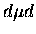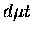Next: Scattering calculations with the Up: Adiabatic approaches Previous: Born's Adiabatic methodThe Dubna group, led by Ponomarev, undertook extensive efforts in the 1970s to solve the muonic three body problem with high accuracy. Their method, known as the Adiabatic Representation method, is based on the expansion of the three-body wave function (Eq. 2.12) in the basis of solutions to the two-centre problem (Eq. 2.13) describing the muonic motion in the field of two fixed nuclei:(26)

whereindicates summation over the discrete states and integration over continuum states, and r and R are given in the co-ordinates shown in Fig. 2.1. The basisis given by solving (in the units)(27)

where the reduced mass is defined with respect to one of the nuclei Ma ():(28)

such that the energyis measured from the ground state of an isolated atom. The right hand side of Eq. 2.13 is replaced byfor the continuum spectrum. The equation for the nuclear wave functionsis given by an infinite set of coupled integro-differentialequations:(29)

where Uij are the effective potentials due to the kinetic energy of the nuclear motion and its coupling to the muon motion, andare the eigenvalues, from which the binding energies are derived. Note that simplified notations given in Ref.  are shown here; for the complete formulae, see a detailed review . Equation 2.15 is similar to the starting point of the Born approximation derivation (Eq. 2.8), but contrary to the approximation made in Eq. 2.10, the non-adiabacity is fully taken into account by the off-diagonal coupling terms Uij.

Ponomarev and his colleagues overcame the computational challenges (and limited computer resources at the time) and solved Eq. 2.15 via systematic expansion in the finite set of the basis functions where the continuum spectra are discretized via:(30)

which gives a truncated system of coupled integro-differential equations with finite number N. In contrast to the Born Adiabatic method, where accuracy was not well determined, the convergence of the expansion can be tested, and the solution was obtained with a controlled accuracy . With a few hundred basis functions, the solution converged, giving an accuracy of 10-1 eV . Thus, they showed for the first time the existence of the loosely bound state for theandmolecules. In fact, for the loosely bound states, contributions from off-diagonal terms from both discrete and continuum states are significant , and this is why earlier adiabatic calculations failed to see such states.Next: Scattering calculations with the Up: Adiabatic approaches Previous: Born's Adiabatic method